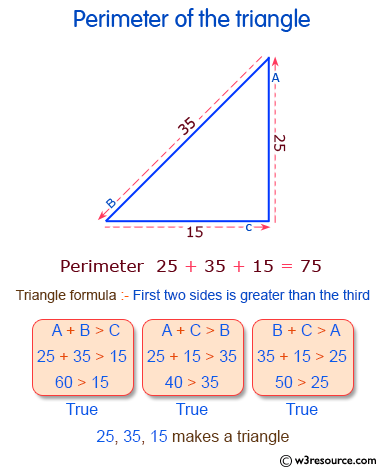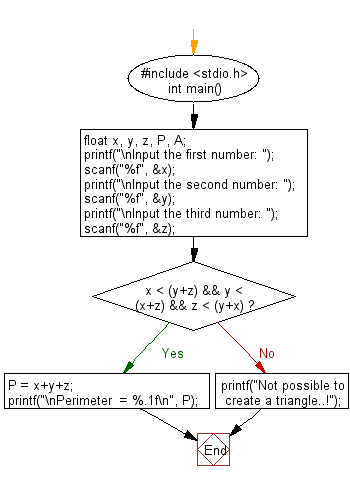﻿ C Program: Calculate the perimeter of the triangle - w3resource# C Exercises: Calculate the perimeter of the triangle

## C Basic Declarations and Expressions: Exercise-23 with Solution

Write a C program that reads three floating values and check if it is possible to make a triangle with them. Also calculate the perimeter of the triangle if the said values are valid.

Pictorial Presentation:C Code:

``````#include <stdio.h>
int main() {
float  x, y, z, P, A;
printf("\nInput the first number: ");
scanf("%f", &x);
printf("\nInput the second number: ");
scanf("%f", &y);
printf("\nInput the third number: ");
scanf("%f", &z);

if(x < (y+z) && y < (x+z) && z < (y+x))
{
P = x+y+z;
printf("\nPerimeter  = %.1f\n", P);

}
else
{
printf("Not possible to create a triangle..!");
}
}
``````

Sample Output:

```Input the first number: 25

Input the second number: 15

Input the third number: 35

Perimeter  = 75.0
```

Flowchart:C Programming Code Editor:

What is the difficulty level of this exercise?

Test your Programming skills with w3resource's quiz.

﻿